## Restrict the domain to find the inverse of a polynomial function

So far, we have been able to find the inverse functions of cubic functions without having to restrict their domains. However, as we know, not all cubic polynomials are one-to-one. Some functions that are not one-to-one may have their domain restricted so that they are one-to-one, but only over that domain. The function over the restricted domain would then have an inverse function. Since quadratic functions are not one-to-one, we must restrict their domain in order to find their inverses.

### A General Note: Restricting the Domain

If a function is not one-to-one, it cannot have an inverse. If we restrict the domain of the function so that it becomes one-to-one, thus creating a new function, this new function will have an inverse.

### How To: Given a polynomial function, restrict the domain of a function that is not one-to-one and then find the inverse.

1. Restrict the domain by determining a domain on which the original function is one-to-one.
2. Replace f(x) with y.
3. Interchange x and y.
4. Solve for y, and rename the function or pair of function ${f}^{-1}\left(x\right)$.
5. Revise the formula for ${f}^{-1}\left(x\right)$ by ensuring that the outputs of the inverse function correspond to the restricted domain of the original function.

### Example 3: Restricting the Domain to Find the Inverse of a Polynomial Function

Find the inverse function of f:

1. $f\left(x\right)={\left(x - 4\right)}^{2}, x\ge 4$
2. $f\left(x\right)={\left(x - 4\right)}^{2}, x\le 4$

### Solution

The original function $f\left(x\right)={\left(x - 4\right)}^{2}$ is not one-to-one, but the function is restricted to a domain of $x\ge 4$ or $x\le 4$ on which it is one-to-one.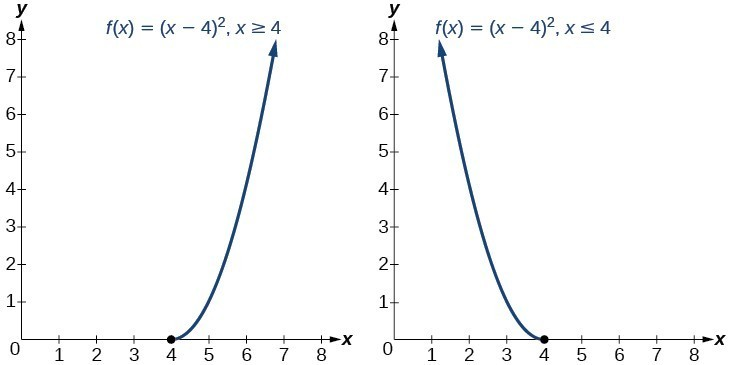Figure 5

To find the inverse, start by replacing $f\left(x\right)$ with the simple variable y.

$\begin{cases}y={\left(x - 4\right)}^{2}\hfill & \text{Interchange } x \text{ and }y.\hfill \\ x={\left(y - 4\right)}^{2}\hfill & \text{Take the square root}.\hfill \\ \pm \sqrt{x}=y - 4 \hfill & \text{Add } 4 \text{ to both sides}.\hfill \\ 4\pm \sqrt{x}=y\hfill & \hfill \end{cases}$

This is not a function as written. We need to examine the restrictions on the domain of the original function to determine the inverse. Since we reversed the roles of x and y for the original f(x), we looked at the domain: the values x could assume. When we reversed the roles of x and y, this gave us the values y could assume. For this function, $x\ge 4$, so for the inverse, we should have $y\ge 4$, which is what our inverse function gives.

1. The domain of the original function was restricted to $x\ge 4$, so the outputs of the inverse need to be the same, $f\left(x\right)\ge 4$, and we must use the + case:
${f}^{-1}\left(x\right)=4+\sqrt{x}$
2. The domain of the original function was restricted to $x\le 4$, so the outputs of the inverse need to be the same, $f\left(x\right)\le 4$, and we must use the – case:
${f}^{-1}\left(x\right)=4-\sqrt{x}$

### Analysis of the Solution

On the graphs below, we see the original function graphed on the same set of axes as its inverse function. Notice that together the graphs show symmetry about the line $y=x$. The coordinate pair $\left(4, 0\right)$ is on the graph of f and the coordinate pair $\left(0, 4\right)$ is on the graph of ${f}^{-1}$. For any coordinate pair, if (a, b) is on the graph of f, then (b, a) is on the graph of ${f}^{-1}$. Finally, observe that the graph of f intersects the graph of ${f}^{-1}$ on the line = x. Points of intersection for the graphs of f and ${f}^{-1}$ will always lie on the line = x.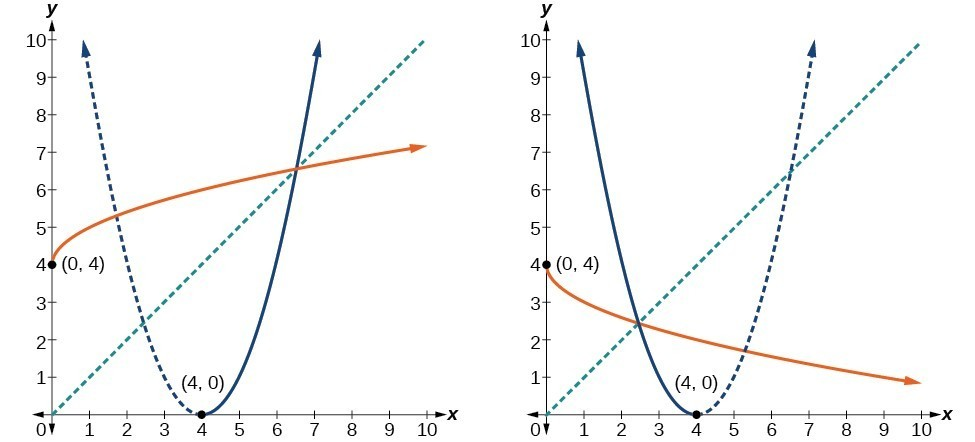Figure 6

### Example 4: Finding the Inverse of a Quadratic Function When the Restriction Is Not Specified

Restrict the domain and then find the inverse of

$f\left(x\right)={\left(x - 2\right)}^{2}-3$.

### Solution

We can see this is a parabola with vertex at $\left(2, -3\right)$ that opens upward. Because the graph will be decreasing on one side of the vertex and increasing on the other side, we can restrict this function to a domain on which it will be one-to-one by limiting the domain to $x\ge 2$.

To find the inverse, we will use the vertex form of the quadratic. We start by replacing f(x) with a simple variable, y, then solve for x.

$\begin{cases}y={\left(x - 2\right)}^{2}-3\hfill & \text{Interchange } x \text{ and } y.\hfill \\ x={\left(y - 2\right)}^{2}-3\hfill & \text{Add 3 to both sides}.\hfill \\ x+3={\left(y - 2\right)}^{2}\hfill & \text{Take the square root}.\hfill \\ \pm \sqrt{x+3}=y - 2\hfill & \text{Add 2 to both sides}.\hfill \\ 2\pm \sqrt{x+3}=y\hfill & \text{Rename the function}.\hfill \\ {f}^{-1}\left(x\right)=2\pm \sqrt{x+3}\hfill & \hfill \end{cases}$

Now we need to determine which case to use. Because we restricted our original function to a domain of $x\ge 2$, the outputs of the inverse should be the same, telling us to utilize the + case

${f}^{-1}\left(x\right)=2+\sqrt{x+3}$

If the quadratic had not been given in vertex form, rewriting it into vertex form would be the first step. This way we may easily observe the coordinates of the vertex to help us restrict the domain.

### Analysis of the Solution

Notice that we arbitrarily decided to restrict the domain on $x\ge 2$. We could just have easily opted to restrict the domain on $x\le 2$, in which case ${f}^{-1}\left(x\right)=2-\sqrt{x+3}$. Observe the original function graphed on the same set of axes as its inverse function in the graph below. Notice that both graphs show symmetry about the line yx. The coordinate pair $\left(2,\text{ }-3\right)$ is on the graph of f and the coordinate pair $\left(-3,\text{ }2\right)$ is on the graph of ${f}^{-1}$. Observe from the graph of both functions on the same set of axes that

$\text{domain of }f=\text{range of } {f}^{-1}=\left[2,\infty \right)$

and

$\text{domain of }{f}^{-1}=\text{range of } f=\left[-3,\infty \right)$

Finally, observe that the graph of f intersects the graph of ${f}^{-1}$ along the line = x.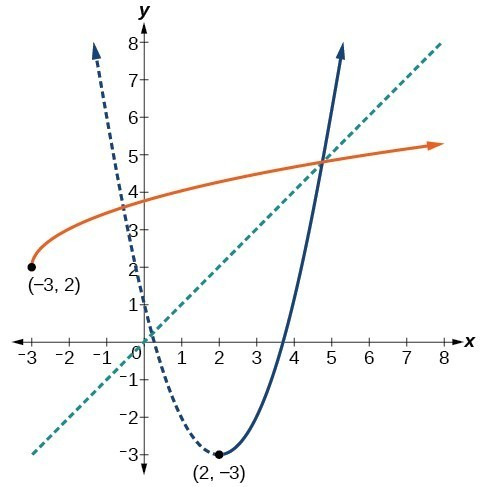Figure 7

### Try It 3

Find the inverse of the function $f\left(x\right)={x}^{2}+1$, on the domain $x\ge 0$.

Solution

## Solving Applications of Radical Functions

Notice that the functions from previous examples were all polynomials, and their inverses were radical functions. If we want to find the inverse of a radical function, we will need to restrict the domain of the answer because the range of the original function is limited.

### How To: Given a radical function, find the inverse.

1. Determine the range of the original function.
2. Replace f(x) with y, then solve for x.
3. If necessary, restrict the domain of the inverse function to the range of the original function.

### Example 5: Finding the Inverse of a Radical Function

Restrict the domain and then find the inverse of the function $f\left(x\right)=\sqrt{x - 4}$.

### Solution

Note that the original function has range $f\left(x\right)\ge 0$. Replace $f\left(x\right)$ with y, then solve for x.

$\begin{cases}\hfill y& =\sqrt{x - 4}& \text{Replace}f\left(x\right)\text{with}y.\\ \hfill x& =\sqrt{y - 4}& \text{Interchange}x\text{and}y.\hfill \\ \hfill x& =\sqrt{y - 4}& \text{Square each side}.\hfill \\ \hfill {x}^{2}& =y - 4& \text{Add 4}.\hfill \\ \hfill {x}^{2}+4& =y& \text{Rename the function}{f}^{-1}\left(x\right).\hfill \\ \hfill {f}^{-1}\left(x\right)& ={x}^{2}+4& \end{cases}$

Recall that the domain of this function must be limited to the range of the original function.

${f}^{-1}\left(x\right)={x}^{2}+4,x\ge 0$

### Analysis of the Solution

Notice in the graph below that the inverse is a reflection of the original function over the line = x. Because the original function has only positive outputs, the inverse function has only positive inputs.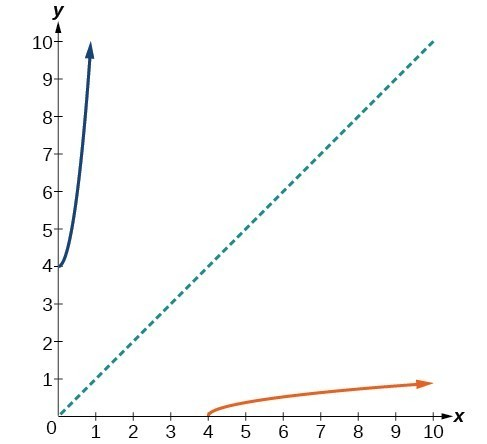Figure 8

### Try It 4

Restrict the domain and then find the inverse of the function $f\left(x\right)=\sqrt{2x+3}$.

Solution

## Solving Applications of Radical Functions

Radical functions are common in physical models, as we saw in the section opener. We now have enough tools to be able to solve the problem posed at the start of the section.

### Example 6: Solving an Application with a Cubic Function

A mound of gravel is in the shape of a cone with the height equal to twice the radius. The volume of the cone in terms of the radius is given by

$V=\frac{2}{3}\pi {r}^{3}$

Find the inverse of the function $V=\frac{2}{3}\pi {r}^{3}$ that determines the volume V of a cone and is a function of the radius r. Then use the inverse function to calculate the radius of such a mound of gravel measuring 100 cubic feet. Use $\pi =3.14$.

### Solution

Start with the given function for V. Notice that the meaningful domain for the function is $r\ge 0$ since negative radii would not make sense in this context. Also note the range of the function (hence, the domain of the inverse function) is $V\ge 0$. Solve for r in terms of V, using the method outlined previously.

$\begin{cases} V=\frac{2}{3}\pi {r}^{3}\hfill & \hfill \\ {r}^{3}=\frac{3V}{2\pi }\hfill & \text{Solve for }{r}^{3}.\hfill \\ r=\sqrt{\frac{3V}{2\pi }}\hfill & \text{Solve for }r.\hfill \end{cases}$

This is the result stated in the section opener. Now evaluate this for V = 100 and $\pi =3.14$.

$\begin{cases}r & =\sqrt{\frac{3V}{2\pi }} \\\text{ } & =\sqrt{\frac{3\cdot 100}{2\cdot 3.14}}\hfill \\\text{ } & \approx \sqrt{47.7707}\hfill \\ \text{ } & \approx 3.63\hfill \end{cases}$

## Determining the Domain of a Radical Function Composed with Other Functions

When radical functions are composed with other functions, determining domain can become more complicated.

### Example 7: Finding the Domain of a Radical Function Composed with a Rational Function

Find the domain of the function $f\left(x\right)=\sqrt{\frac{\left(x+2\right)\left(x - 3\right)}{\left(x - 1\right)}}$.

### Solution

Because a square root is only defined when the quantity under the radical is non-negative, we need to determine where $\frac{\left(x+2\right)\left(x - 3\right)}{\left(x - 1\right)}\ge 0$. The output of a rational function can change signs (change from positive to negative or vice versa) at x-intercepts and at vertical asymptotes. For this equation, the graph could change signs at x = –2, 1, and 3.

To determine the intervals on which the rational expression is positive, we could test some values in the expression or sketch a graph. While both approaches work equally well, for this example we will use a graph.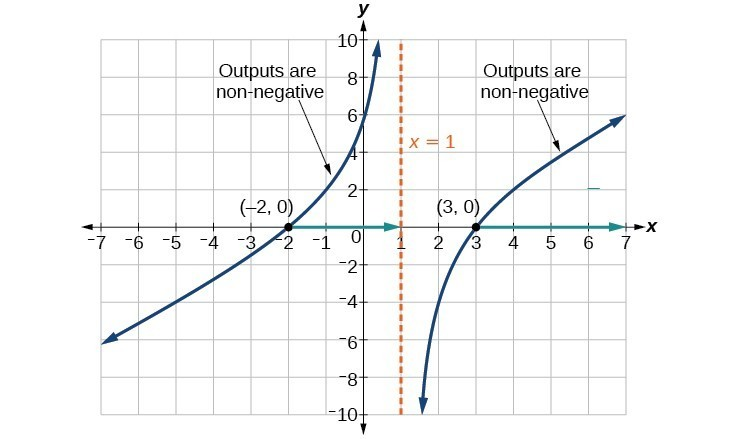Figure 9

This function has two x-intercepts, both of which exhibit linear behavior near the x-intercepts. There is one vertical asymptote, corresponding to a linear factor; this behavior is similar to the basic reciprocal toolkit function, and there is no horizontal asymptote because the degree of the numerator is larger than the degree of the denominator. There is a y-intercept at (0, 6).

From the y-intercept and x-intercept at = –2, we can sketch the left side of the graph. From the behavior at the asymptote, we can sketch the right side of the graph.

From the graph, we can now tell on which intervals the outputs will be non-negative, so that we can be sure that the original function f(x) will be defined. f(x) has domain $-2\le x<1\text{or}x\ge 3$, or in interval notation, $\left[-2,1\right)\cup \left[3,\infty \right)$.

## Finding Inverses of Rational Functions

As with finding inverses of quadratic functions, it is sometimes desirable to find the inverse of a rational function, particularly of rational functions that are the ratio of linear functions, such as in concentration applications.

### Example 8: Finding the Inverse of a Rational Function

The function $C=\frac{20+0.4n}{100+n}$ represents the concentration C of an acid solution after n mL of 40% solution has been added to 100 mL of a 20% solution. First, find the inverse of the function; that is, find an expression for n in terms of C. Then use your result to determine how much of the 40% solution should be added so that the final mixture is a 35% solution.

### Solution

We first want the inverse of the function. We will solve for n in terms of C.

$\begin{cases}C=\frac{20+0.4n}{100+n}\\ C\left(100+n\right)=20+0.4n\\ 100C+Cn=20+0.4n\\ 100C - 20=0.4n-Cn\\ 100C - 20=\left(0.4-C\right)n\\ n=\frac{100C - 20}{0.4-C}\end{cases}$

Now evaluate this function for C=0.35 (35%).

$\begin{cases}n=\frac{100\left(0.35\right)-20}{0.4 - 0.35}\\ =\frac{15}{0.05}\\ =300\end{cases}$

We can conclude that 300 mL of the 40% solution should be added.

### Try It 5

Find the inverse of the function $f\left(x\right)=\frac{x+3}{x - 2}$.

Solution